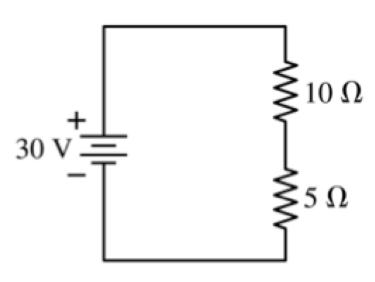# Problem: The potential difference across the 10 resistor is A. 30 V. B. 20 V. C. 15 V. D. 10 V. E. 5 V.

###### FREE Expert Solution

Ohm's law:

$\overline{){\mathbf{V}}{\mathbf{=}}{\mathbf{I}}{\mathbf{R}}}$

Series equivalent resistance:

$\overline{){{\mathbf{R}}}_{\mathbf{e}\mathbf{q}}{\mathbf{=}}{{\mathbf{R}}}_{{\mathbf{1}}}{\mathbf{+}}{{\mathbf{R}}}_{{\mathbf{2}}}}$

Req = R1 + R2 = 10 + 5 = 15Ω

ICIRCUIT  = V/Req = 30/15 = 2 A

93% (363 ratings)###### Problem Details

The potential difference across the 10 resistor isA. 30 V.

B. 20 V.

C. 15 V.

D. 10 V.

E. 5 V.